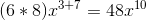# Pre-Algebra : Product Rule of Exponents

## Example Questions

### Example Question #31 : Product Rule Of Exponents

Simplify: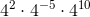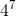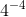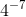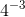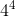Explanation:

Since the bases are all alike and are multiplied, the exponents can be added.

In mathematical terms: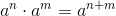Plugging in four as our base and the various exponents we get the following,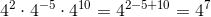.

### Example Question #32 : Product Rule Of Exponents

Expand: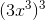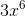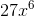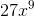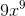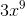Explanation:

The exponents can be multiplied in this case.

Expand out the terms to verify.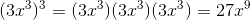### Example Question #31 : Product Rule Of Exponents

Solve: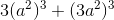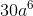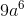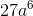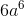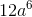Explanation:

Evaluate the quantities inside the parentheses first. The powers are multiplied when they are bounded in the parentheses.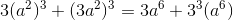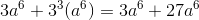Combine like-terms.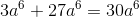### Example Question #32 : Product Rule Of Exponents

Evaluate: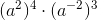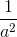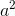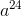Explanation:

Simplify each parentheses before multiplying.

Recall the rules of exponents which states if exponents are being raised to another one they are multiplied and if like bases are being multiplied their exponents are added.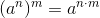Applying these rules we get,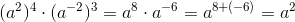.

### Example Question #131 : Polynomials

Simplify: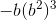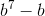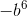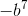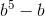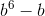Explanation:

Evaluate the powers by multiplication.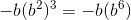Use the power rule to combine the terms.  The exponents can then be added.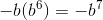### Example Question #131 : Polynomials

Solve: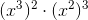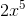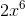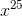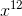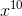Explanation:

Simplify the first two terms using the product rule of exponents.  When the base of some power is squared, we can simply multiply the two powers together.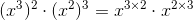Simplify the powers.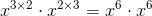Use the power rule to add the powers since the bases are alike.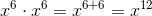### Example Question #132 : Polynomials

Solve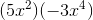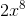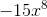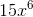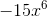Explanation:

If a variable has an exponent with the value of a, it means we are taking that variable and mulitplying it by itself a times.  For example,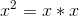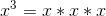and so on.

So when we multiplywe can expand it to look like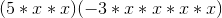If we multiply, we get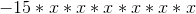which equals### Example Question #134 : Polynomials

Simplify the following: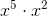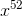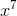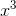Explanation:

When you multiply two terms together with the same base, you add the exponents.  So,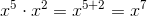### Example Question #133 : Polynomials

Simplify the following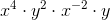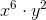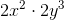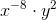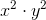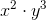Explanation:

When multiplying terms with the same base, we add the exponents.  Therefore,can be written as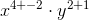### Example Question #134 : Polynomials

Simplify the following expression: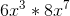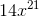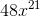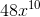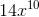Explanation:

Simplify the following expression:So, we are multiplying exponents. When doing so, we need to treat the numbers out in front like regular multiplication, and add the exponents: